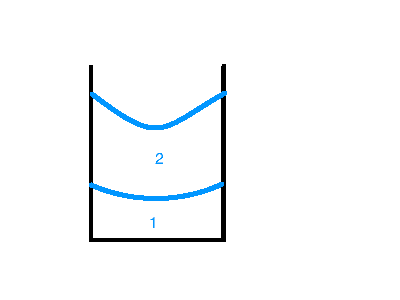# Surface of 2 rotating fluids in a cylindrical container

## Homework Statement

In a cylindrical container (with radius R) there are 2 fluids (separated like water and oil, fluid 1 lies under fluid 2) with given volumes V_i, given densities ρ_i.
You let them rotate with respective angular frequencies ω_i.
There is no friction.
Find the functions of form $y_i=a_i x^2+b_i$ (cylindrical coordinates) that describe the surface (y_i) of each of the 2 fluids.
[Ignore cases of the surfaces touching each other or the bottom of the container]

2. The attempt at a solution
I was thinking about finding the functions y_i that would minimize the overall energy for the given values.
I wrote down the following:
$E=E_{pot1}+E_{pot2}+E_{rot1}+E_{rot2}$
This is a function of ρ, ω, V, a_1, b_1, a_2, b_2
But because Volume is conserved, b_i depends on a_i so we can leave out b_i as an argument of the function.
Now we want to minimize the energy by solving the following two equations for the 2 unknown a.
$\frac{∂E}{a_1}=0$
$\frac{∂E}{a_2}=0$
By solving this we obtain a_i --> b_i --> y_i(r)

Is "minimizing the energy" a legitimate way of finding a solution for this problem?
I'm being careful, because I tried this on the same problem but exclusively for 1 fluid and it didn't work, because ∂E/∂a was independent of a.

just in case you need some visualizationI've as far as to see, that my current approach also contradicts the observation that the fluids will go to the walls of the container for faster rotations.
This leads me to thinking that i will definitely have to work in the centripetal force.
Maybe one would have to add E=∫dE=∫F_cent*dr to the energy (following from F=dE/dr). This is just a wild guess.
Help is very much appreciated!